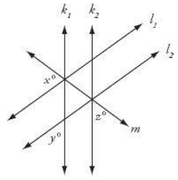• NEW! FREE Beat The GMAT QuizzesHundreds of Questions Highly Detailed Reporting Expert Explanations
• 7 CATs FREE!
If you earn 100 Forum Points

Engage in the Beat The GMAT forums to earn
100 points for $49 worth of Veritas practice GMATs FREEVERITAS PRACTICE GMAT EXAMS Earn 10 Points Per Post Earn 10 Points Per Thanks Earn 10 Points Per Upvote ## In the figure above, lines k1 and k2 are parallel to each tagged by: BTGmoderatorLU ##### This topic has 1 expert reply and 1 member reply ### Top Member ## In the figure above, lines k1 and k2 are parallel to each## Timer 00:00 ## Your Answer A B C D E ## Global Stats Difficult Source: Manhattan PrepIn the figure above, lines k1 and k2 are parallel to each other, lines l1 and l2 are parallel to each other, and line m passes through the intersection points of k1 with l1 and k2 with l2. What is the value of x? 1) x = 3z - y 2) (y - z)^2 = 225 The OA is E. ### GMAT/MBA Expert GMAT InstructorJoined 22 Aug 2016 Posted: 2008 messages Followed by: 31 members Upvotes: 470 BTGmoderatorLU wrote: Source: Manhattan PrepIn the figure above, lines k1 and k2 are parallel to each other, lines l1 and l2 are parallel to each other, and line m passes through the intersection points of k1 with l1 and k2 with l2. What is the value of x? 1) x = 3z - y 2) (y - z)^2 = 225 The OA is E. In the triangle enclosed by angles x, y and z, we have angles: (180 - x), y, and (180 - z). Thus, the sum of all the three angles of the triangle = (180 - x) + y + (180 - z) = 180 => x + y + z = 180. Question: What's the value of x? Let's take each statement one by one. 1) x = 3z - y Plugging-in the value of x in x + y + z = 180, we get (3z - y) + y + z = 180 => z = 45º Can't get the value of x. Insufficient. 2) (y - z)^2 = 225 => y - z = 15 or y - z = -15 Even with the help of x + y + z = 180, we can't get x. Insufficient. (1) and (2) Case 1: y - z = 15 => At z = 45, we have y - z = 15 => y = 60; thus, x + y + z = 180 => x + 60 + 45 = 180 => x = 75 Case 2: y - z = -15 => At z = 45, we have y - z = -15 => y = 30; thus, x + y + z = 180 => x + 30 + 45 = 180 => x = 105 No unique value of x. Insufficient. The correct answer: E Hope this helps! -Jay _________________ Manhattan Review GMAT Prep Locations: Manhattan Review Mehdipatnam | Hyderabad GMAT Prep | Bangalore GMAT Courses | Dilsukhnagar GRE Prep | and many more... Schedule your free consultation with an experienced GMAT Prep Advisor! Click here. ### Top Member Legendary MemberJoined 02 Mar 2018 Posted: 1173 messages Followed by: 2 members We are finding the value of x angle z are corresponding angles, the same thing goes for y angle y are corresponding angles at the intersection of line $$k_1$$ ; $$l_1$$ $$and\ \ m_1$$ $$angles\ \left(x+y+2\right)=180\deg ree\left(angle\ on\ a\ straight\ line\right)$$ statement 1 $$x=3z-y$$ we know that $$x+y+z=180\ and\ x=3z-y$$ $$3z-y+y+z=180$$ $$\frac{4z}{4}=\frac{180}{4}$$ $$z=45$$ but x is still unknown. Hence statement 1 is INSUFFICIENT statement 2 $$\left(y-2\right)^2=225$$ square rooting both sides $$\sqrt{\left(y-2\right)^2}=\sqrt{225}$$ $$y-2=\pm15$$ We cannot determine the value of x with the information provided in statement 2 Hence 2 is INSUFFICIENT. Combining statement 1 and 2 together $$z=45\ and\ y-z=\pm15$$ $$y=60\deg ree\ \ OR\ y=30\deg ree$$ $$x+y+z=180\deg ree$$ $$x=180-y-z$$ $$x=180-45-60\ \ OR\ x=180-45-30$$ $$x=75\ \ O\ R\ x=105$$ There are more than one solution Hence the two statements together are not SUFFICIENT. $$answer\ is\ option\ E$$ •Free Practice Test & Review How would you score if you took the GMAT Available with Beat the GMAT members only code •5 Day FREE Trial Study Smarter, Not Harder Available with Beat the GMAT members only code •Free Trial & Practice Exam BEAT THE GMAT EXCLUSIVE Available with Beat the GMAT members only code •Magoosh Study with Magoosh GMAT prep Available with Beat the GMAT members only code •Get 300+ Practice Questions 25 Video lessons and 6 Webinars for FREE Available with Beat the GMAT members only code •Award-winning private GMAT tutoring Register now and save up to$200

Available with Beat the GMAT members only code

•1 Hour Free
BEAT THE GMAT EXCLUSIVE

Available with Beat the GMAT members only code

•5-Day Free Trial
5-day free, full-access trial TTP Quant

Available with Beat the GMAT members only code

•FREE GMAT Exam
Know how you'd score today for \$0

Available with Beat the GMAT members only code

•Free Veritas GMAT Class
Experience Lesson 1 Live Free

Available with Beat the GMAT members only code

### Top First Responders*

1Ian Stewart 44 first replies
2Brent@GMATPrepNow 33 first replies
3Scott@TargetTestPrep 32 first replies
4Jay@ManhattanReview 24 first replies
5GMATGuruNY 18 first replies
* Only counts replies to topics started in last 30 days
See More Top Beat The GMAT Members

### Most Active Experts

1Scott@TargetTestPrep

Target Test Prep

149 posts
2Max@Math Revolution

Math Revolution

93 posts
3Ian Stewart

GMATiX Teacher

53 posts
4Brent@GMATPrepNow

GMAT Prep Now Teacher

50 posts
5GMATGuruNY

The Princeton Review Teacher

30 posts
See More Top Beat The GMAT Experts